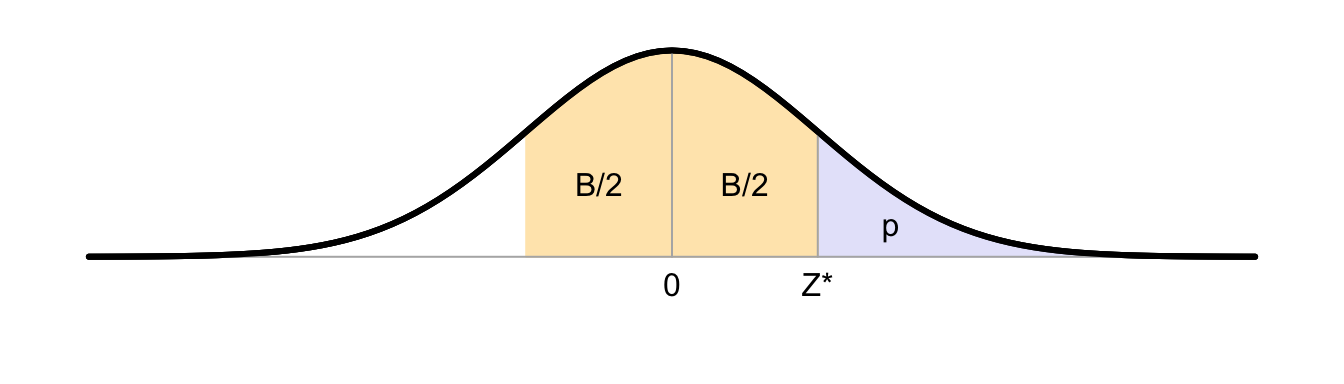# B Standard normal probability distributionThe critical value $$Z^*$$ given below has a probability of $$p$$ under $$H_0$$, i.e., $$P(Z > Z^*|H_0)=p$$ (the blue area), and it has a probability of $$B$$ to have a value in the interval $$(-Z^*, +Z^*)$$ (the yellow area). The $$Z$$ distribution is symmetrical around $$Z=0$$, hence $$P(Z < -Z^*) = P(Z > Z^*)$$.

The first table reports the critical boundary values $$Z^*$$ for some frequently used probabilities of $$p$$ and frequently used confidence intervals of $$B$$:

p 0.2 0.1 0.05 0.025 0.01 0.005 0.0025 0.001
B 60% 80% 90% 95% 98% 99% 99.5% 99.8%
Z* 0.8416 1.282 1.645 1.960 2.326 2.576 2.807 3.090

The second table reports the probabilities $$p$$ and confidence intervals $$B$$ for some frequently used critical values of $$Z^*$$:

p 0.3085 0.1587 0.0668 0.0228 0.0062 0.0013 0.0002
B 38.29% 68.27% 86.64% 95.45% 98.76% 99.73% 99.95%
Z* 0.5 1 1.5 2 2.5 3 3.5